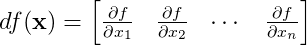# Notes on Vector Calculus

Published:

This post contains some of the important notes which come in handy while working with vector-calculus.

## Vector Space

A vector space is a collection of objects called vectors, which may be added together and multiplied/scaled by scalars. Scalars are often taken to be real numbers.

$\mathbf{x} = \begin{bmatrix} x_{1} \\ x_{2} \\ \vdots \\ x_{n} \end{bmatrix}$

$\mathbf{x}$ is a vector of $n$ dimensions.

## Function

A function is relationship between two sets. It associates an element from frist set to exactly one element of second set.

$f: \mathit{X} \rightarrow \mathit{Y} \label{eq1}$ $\mathbf{x} \mapsto f(\mathbf{x}) \label{eq2}$

In equation \eqref{eq1}, the set represented by $\mathit{X}$ is called domain of function $f$ and $\mathit{Y}$ is called codomain of function $f$. This notation can be read as ‘the function $f$ mapping elements of set $\mathit{X}$ to elements of set $\mathit{Y}$’. Similarly, \eqref{eq2} can be read as ‘$f$ maps $\mathbf{x}$ to $f(\mathbf{x})$’.

## Scalar-valued function or Scalar field

The function which maps a vector to a scalar value.

$f:\Re^n \rightarrow \Re \label{eq3}$ $y = f(\mathbf{x}) \label{scalar-func}$

Equation \eqref{eq3} maps an $n$-dimensional vector to a scalar value. It is a scalar-valued function. $y$ is a scalar and $\mathbf{x}$ is a vector of $n$-dimensions in \eqref{scalar-func}.

## Vector-valued function

A vector-valued function maps one vector space to another vector space.

$\mathbf{f}: \Re^n \rightarrow \Re^m \label{eq4}$ $\mathbf{y} = \mathbf{f}(\mathbf{x}) \label{eq5}$

Equation \eqref{eq4} maps an $n$-dimensional vector to a vector of $m$-dimensions. It is a vector-valued function. The output value $\mathbf{y}$ in \eqref{eq5} is of $m$-dimensions and the corresponding input value $\mathbf{x}$ is of $n$-dimensions.

The gradient of a scalar-valued differentiable function is also referred to as vector field or vector-valued function.

$\bigtriangledown f: \Re^n \rightarrow \Re^n \label{eq-grad1}$

Gradient of vector-valued function at a point $\mathbf{x}$ in domain $\mathit{X} \in \Re^n$ is:

$\bigtriangledown f(\mathbf{x}) = \begin{bmatrix} \frac{\partial f}{\partial x_{1}} \\ \frac{\partial f}{\partial x_{2}} \\ \vdots \\ \frac{\partial f}{\partial x_{n}} \end{bmatrix}\label{eq-grad2}$

At each point of a scalar-valued function, a gradient is a tangent vector representing an infinitesimal change in vector input. Notice that here a column vector is used to represent the gradient of the function at point $x$.

## Derivative

Derivative at each point of the scalar-valued function is a co-tangent vector, a linear form that expresses how much the scalar output of a function changes for a given infinitesimal change in the input vector. Notice, we represent the derivative of a scalar-valued function as a row vector. This is unlike the gradient vector (that used column vector).$\label{eq-derivative1}$

Note: derivative is just a transpose of gradient.

$df(\mathbf{x}) = \bigtriangledown f(\mathbf{x})^{T} \label{eq-derivative2}$

## Linear approximation of a scalar-valued function

Linear approximation of a function $f(\mathbf{x})$ at a point $\mathbf{x_{0}} \in \Re^{n}$:

$f(\mathbf{x}) \approx f(\mathbf{x_0}) + (\bigtriangledown f)_{\mathbf{x_0}} (\mathbf{x} - \mathbf{x_0})$

## Jacobian (a.k.a. derivative of Vector-valued Function)

Derivative of $\mathbf{f}$ in equation \eqref{eq5} linearly maps tangent space $T_{\mathbf(x)}$ to tanget space $T_{\mathbf(y)}$.

First order-partial derivative of vector-valued function forms the Jacobian matrix. We will denote the Jacobian by the notation $\mathbf{J}$

Note: Jacobian has the dimensions of $m \times n$.

A Jacobian is the vertical stack of derivative vectors corresponding to each output element of vector $\mathbf{y}$ (i.e., row of the Jacobian matrix). This definition makes sense, and we can relate it to the derivative of a scalar-valued function defined above. The derivative of a scalar-valued function, $m=1$, is a row vector.

## Hessian matrix

Hessian matrix for scalar-valued function or scalar field given by \eqref{scalar-func} is a square matrix of second order partial derivatives of this scalar-valued function.

Tags: